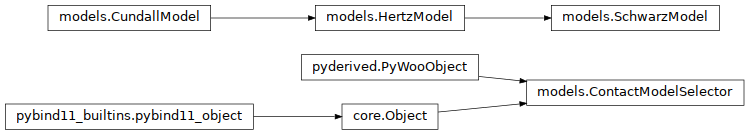# woo.models¶Collection of classes providing python-only implementation of various implementation models, mostly for testing or plotting in documentation. Plus a class for selecting material model along with all its parameters for use in preprocessors.

class woo.models.CundallModel(r1, r2, E1, E2=None, ktDivKn=0.2, name='')[source]

Linear model [CS79].

Initialize the model with constants. If E2 is not given, it is the same as E1

F_u()[source]

Return $$F=k_n u_N$$ (uses the Woo convention).

static make_plot()[source]
class woo.models.HertzModel(r1, r2, E1, nu1, E2=None, nu2=None, name='')[source]

Purely elastic Hertz contact model; base class for SchwarzModel. For details, see Hertzian contact models.

Initialize the model with constants. If nu2 is not given, it is the same as nu1. Passes most parameters to CundallModel.

F_a(a)[source]

$$F(a)$$

F_delta(delta)[source]

$$F(\\delta)$$

a_F(F)[source]

$$a(F)$$

a_delta(delta)[source]

$$a(\delta)=\sqrt{R\delta}$$

delta_a(a)[source]

$$\delta(a)$$

class woo.models.SchwarzModel(r1, r2, E1, nu1, alpha, gamma, E2=None, nu2=None, name='')[source]

Schwarz contact model, following [Sch03]. Details are given in Adhesive models.

Initialize the model; passes elastic parameters to HertzModel.

F_a(a)[source]

$$F(a)$$

aHat(a)[source]

$$\hat a(a)$$

aHat_fHat(fHat)[source]
aHi(delta)[source]

$$a_{\max}=a_{\min}+\sqrt{R(\delta-\delta_{\min})}$$.

aMin()[source]

$$a_{\min}=(R\xi)^\frac{2}{3}$$.

aUnhat(aHat)[source]

$$a(\hat a)$$

a_F(F)[source]

$$a(F)$$

$$a(\delta)$$

deltaHat(delta)[source]

$$\hat\delta(\delta)$$

deltaHat_aHat(aHat)[source]
deltaMin()[source]

$$\delta_{\min}=-3R^\frac{1}{3}\xi^\frac{4}{3}$$.

deltaUnhat(deltaHat)[source]

$$\delta(\hat \delta)$$

delta_a(a)[source]

$$\delta(a)$$

fHat(F)[source]

$$\hat F(F)$$

fHat_aHat(aHat)[source]
fUnhat(fHat)[source]

$$F(\hat F)$$

static makeDefault(**kw)[source]

Return instance of the model with some “reasonable” parameters, which are overridden with **kw. Useful for quick testing.

static normalized_plot(what, alphaGammaName, N=1000, stride=50, aHi=[], legendAlpha=0.5)[source]

Create normalized plot as it appears in [Mau92] including range of axes with normalized quantities. This function is mainly useful for documentation of Woo itself.

Parameters
• what – one of a(delta), F(delta), a(F)

• alphaGammaName – list of (alpha,gamma,name) tuples which are passed to the SchwarzModel constructor

• N – numer of points in linspace for each axis

• stride – stride for plotting inverse relationships (with points)

• aHi – with what==a(delta), show upper bracket for $a$ for i-th model, if i appears in the list

class woo.models.ContactModelSelector(self: woo.core.Object) None[source]

User interface for humanely selecting contact model and all its features, plus functions returning respective woo.dem.CPhysFunctor and woo.dem.LawFunctor objects.

name(= 'linear')

Material model to use.

[type: str, choices: linear, pellet, Hertz, DMT, Schwarz, concrete, ice, luding]

numMat(= Vector2i(1, 1))

Minimum and maximum number of material definitions.

[type: Vector2i, not shown in the UI, read-only in python]

matDesc(= [])

List of strings describing individual materials. Keep the description very short (one word) as it will show up in the UI combo box for materials.

[type: [str, …], not shown in the UI]

mats(= [<FrictMat @ 0x3b903d0>])

Material definitions

[type: [Material, …]]

distFactor(= 1.0)

Distance factor for sphere-sphere contacts (copied to woo.dem.DemField.distFactor)

[type: float]

poisson(= 0.2)

Poisson ratio (woo.dem.Cp2_HertzMat_HertzPhys.poisson)

[type: float]

restitution(= 1.0)

Restitution coefficient for models with viscosity (woo.dem.Cp2_FrictMat_HertzPhys.en).

[type: float]

damping(= 0.2)

Numerical (non-viscous) damping (woo.dem.Leapfrog.damping).

Note

This damping value is only used for linear and ice mocel, otherwise the model has its own damping and this value will be ignored (woo.dem.Leapfrog.damping will be zero).

[type: float]

linRoll(= False)

Linear model: enable rolling, with parameters set in linRollParams.

[type: bool]

linRollParams(= Vector3(1, 1, 1))

Rolling parameters for the linear model, in the order of relRollStiff, relTwistStiff, rollTanPhi.

[type: Vector3]

plastSplit(= False)

Split plastic dissipation into the normal and tangent component (obj:woo.dem.Law2_L6Geom_PelletPhys_Pellet.plastSplit).

[type: bool]

pelletThin(= Vector6(0, 0, 0, 0, 0, 0))

Pellet model: parameters for plastic thinning (decreasing pellet radius during normal plastic loading); their order is thinRate, thinRelRMin, thinExp, thinRefRad, thinMinExp, thinRateExp.

[type: Vector6]

pelletConf(= Vector3(0, 0, 0))

Pellet model: parameters for history-independent adhesion (“confinement”); the values are confSigma, confRefRad and confExp.

[type: Vector3]

alpha(= 0.0)

Detect whether deprecated alpha is used.

[type: float, not shown in the UI]

surfEnergy(= 0.0)

Detect whether deprecated surfEnergy is used.

[type: float, not shown in the UI]

static __new__(klass, **kw)[source]
__repr__(self: woo.core.Object) str
__str__(self: woo.core.Object) str
deepcopy(**kw)

The c++ dedepcopy uses boost::serialization, we need to use pickle. As long as deepcopy is called from python, this function gets precedence over the c++ one. Additional keyword parameters are used to immediately set parameters on the copy before returning.

getFunctors()[source]

Return tuple of ([CPhysFunctor,...],[LawFunctor,...]) corresponding to the selected model and parameters.

getMat()[source]

Return default-initialized material for use with this model.

getMatClass()[source]

Return class object of material for use with this model.

getNonviscDamping()[source]

Return the value for woo.dem.Leapfrog.damping; returns zero for models with internal damping, and damping for the linear (Linear (Cundall) contact model) and ice (Ice contact model) models.